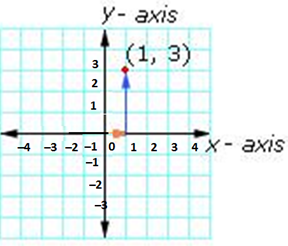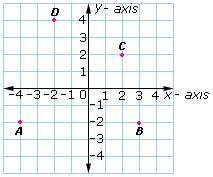Definition Of X-Coordinate

The first number in an ordered pair is the x-coordinate

Another name for x-coordinate of a point is "abscissa"
The x-coordinate of a point tells how far to move left or right from the origin along the x-axis

Examples of X-Coordinate

In the ordered pair (2, 4), the x-coordinate or abscissa is 2
In the ordered pair (- 1, 7), the x-coordinate or abscissa is - 1
To reach the point (1, 3), move 1 unit to the left from the origin and then 3 units upSolved Example on X-Coordinate

Ques: Which of the points has - 4 as the x-coordinate?A. D
B. C
C. B
D. A